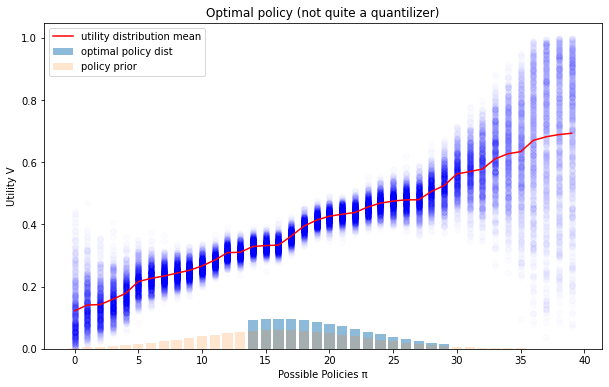# Jeremy Gillen answers Why don’t quantilizers also cut off the upper end of the distribution?

• Quantilizing can be thought of as maximizing a lower bound on the expected true utility, where you know that your true utility is close to your proxy utility function in some region , such that . If we shape this closeness assumption a bit differently, such that the approximation gets worse faster, then sometimes it can be optimal to cut off the top of the distribution (as I did here, see some of the diagrams for quantilizers with the top cut off, I’ll paste one below).The reason normal quantilizers don’t do that is that they are minimizing the distance between and the action distribution, by a particular measure that falls out of the proof (see above link), which allows the lower bound to be as high as possible. Essentially it’s minimizing distribution shift, which allows a better generalization bound.

I think this distribution shift perspective is one way of explaining why we need randomization at all. A delta function is a bigger distribution shift than a distribution that matches the shape of .
But the next question is why are we even in a situation where we need to deal with the worst case across possible true utility functions? One story is that we are dealing with an optimizer that is maximizing trueutility + error, and one way to simplify that is to model it as max min trueutility—error, where the min only controls the error function within the restrictions of the known bound.

I’m not currently super happy with that story and I’m keen for people to look for alternatives, or variations of soft optimization with different types of knowledge about the relationship between the proxy and true utility. Because intuitively it does seem like taking the 99%ile action should be fine under slightly different assumptions.

One example of this is if we know that , where is some heavy tailed noise, and we know the distribution of (and ), then we can calculate the actual optimal percentile action to take, and we should deterministically take that action. But this is sometimes quite sensitive to small errors in our knowledge about the distribution of and particularly . My AISC team has been testing scenarios like this as part of their research.